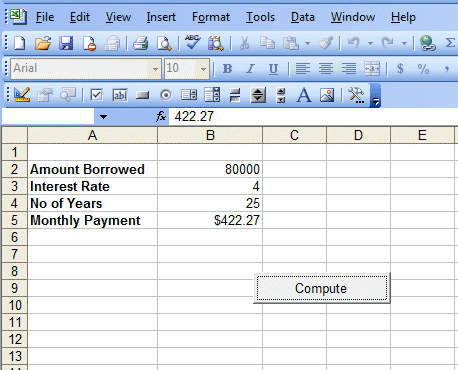Creating a Financial Calculator Using Excel VBA Built-in Function

[Back to Excel VBA Sample Code]

In the previous chapter, we have shown you how to program  a VBA program  that can calculate monthly payment for a loan taken by a borrower. In this example, the financial VBA calculator is doing the same job but we use the built-in worksheet function, PMT. It is very much easier to program than the previous one. The syntax of this function is

WorksheetFunction.pmt (rate, N, amount) where rate is the interest rate, N is the period of payments (of number of periodic payments) and amount is the amount borrowed.

Here is the Code:

 Private Sub CommandButton1_Click() Dim rate, N As Integer Dim amt, payment As Double amt = Cells(2, 2) rate = (Cells(3, 2) / 100) / 12 N = Cells(4, 2) * 12 payment = WorksheetFunction.pmt(rate, N, -amt) Cells(5, 2) = Format(payment, "\$##,###.00") End Sub

Explanation:

As people tend key in the annual interest rate as an integer rather than in decimal form, so we need to divide the rate by 100 and then divide it again by 12 to get the monthly rate.

We put a negative sign in front of the amount borrowed because this is the amount the borrower owed the financial institute, so it should be negative. If we don't put negative, the payment will have a negative sign.Figure 15.1